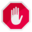## Name

ST_CollectionExtract — Given a (multi)geometry, return a (multi)geometry consisting only of elements of the specified type.

## Synopsis

`geometry ST_CollectionExtract(`geometry collection`)`;

`geometry ST_CollectionExtract(`geometry collection, integer type`)`;

## Description

Given a geometry collection, return a homogeneous multi-geometry. If the type is specified, return a multi-geometry containing only that type, and an EMPTY multigeometry otherwise. If the type is not specified, return a multi-geometry containing only geometries of the highest coordinate dimension. So polygons are preferred over lines, which are preferred over points. Sub-geometries that are not the desired type are ignored. If there are no sub-geometries of the right type, an EMPTY geometry will be returned.

Only points, lines and polygons are supported. The type numbers are:

• 1 == POINT

• 2 == LINESTRING

• 3 == POLYGON

Availability: 1.5.0Prior to 1.5.3 this function returned non-collection inputs untouched, no matter type. In 1.5.3 non-matching single geometries result in a NULL return. In 2.0.0 every case of missing match results in a typed EMPTY return.When specifying 3 == POLYGON a multipolygon is returned even when the edges are shared. This results in an invalid multipolygon for many cases such as applying this function on an ST_Split result.

## Examples

```-- Constants: 1 == POINT, 2 == LINESTRING, 3 == POLYGON
SELECT ST_AsText(ST_CollectionExtract(ST_GeomFromText('GEOMETRYCOLLECTION(GEOMETRYCOLLECTION(POINT(0 0)))'),1));
st_astext
---------------
MULTIPOINT(0 0)
(1 row)

SELECT ST_AsText(ST_CollectionExtract(ST_GeomFromText('GEOMETRYCOLLECTION(GEOMETRYCOLLECTION(LINESTRING(0 0, 1 1)),LINESTRING(2 2, 3 3))'),2));
st_astext
---------------
MULTILINESTRING((0 0, 1 1), (2 2, 3 3))
(1 row)
```